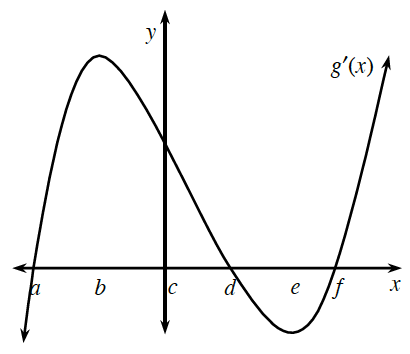### Home > APCALC > Chapter 5 > Lesson 5.1.4 > Problem5-43

5-43.

Using the graph of the slope function at right, determine where the following situations occur for $g$ :

Notice that this is a graph of $g^\prime\left(x\right)$, but you are being asked about $y = g\left(x\right)$.

1. Relative minima.

Relative minima and maxima can exist when $g^\prime\left(x\right) = 0$.
This occurs when $x = a, x = d$, and $x = f$. But which points indicate a maximum and which indicate a minimum?

A minimum occurs when the slope changes from negative to positive.

1. Relative maxima.

See the hint in part (a). A maximum occurs when the slope changes from positive to negative.

1. Interval(s) over which $g$ is increasing.

$g$ is increasing when $g^\prime(x)>0$.

1. Inflection points.

Inflection points can happen where $g^{\prime \prime}\left(x\right) = 0$. Remember, we are looking at the graph of $y = g^\prime\left(x\right)$.

1. Interval(s) over which $g$ is concave up.

$g$ is concave up when $g^{\prime \prime}\left(x\right) > 0$.

1. Interval(s) over which $g$ is concave down.

$g$ is concave down when $g^\prime\left(x\right)$ is decreasing.